6250.0 - Characteristics of Recent Migrants, Australia, Nov 2013
ARCHIVED ISSUE Released at 11:30 AM (CANBERRA TIME) 30/06/2014
 Page tools: .mffeedback,#pjs { display :none; } Enable Javascript to Print PagesPrint All Explanatory Notes Glossary Abbreviations Quality Declaration - Summary Data Quality (Technical Note) TECHNICAL NOTE DATA QUALITY RELIABILITY OF THE ESTIMATES 1 Since the estimates in this publication are based on information obtained from a sample, they are subject to sampling variability. That is, they may differ from those estimates that would have been produced if all dwellings had been included in the survey. One measure of the likely difference is given by the standard error (SE), which indicates the extent to which an estimate might have varied by chance because only a sample of dwellings (or households) was included. There are about two chances in three (67%) that a sample estimate will differ by less than one SE from the number that would have been obtained if all dwellings had been included, and about 19 chances in 20 (95%) that the difference will be less than two SEs. 2 Another measure of the likely difference is the relative standard error (RSE), which is obtained by expressing the SE as a percentage of the estimate: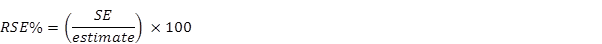3 RSEs for the 2013 Characteristics of Recent Migrants Survey have been calculated using the Jackknife method of variance estimation. This involves the calculation of 30 'replicate' estimates based on 30 different sub samples of the obtained sample. The variability of estimates obtained from these sub samples is used to estimate the sample variability surrounding the estimate. 4 RSEs of all of the estimates in this publication are included in the Data Cube released as part of the publication and are available from the Downloads tab of the publication. 5 Only estimates (numbers and proportions) with RSEs less than 25% are considered sufficiently reliable for most purposes. Estimates with RSEs between 25% and 50% are presented with a cell comment to indicate they are subject to high sample variability and should be used with caution. Estimates with RSEs greater than 50% are presented with a cell comment to indicate that they are considered too unreliable for general use. All cells in the Data Cube with an RSE greater than 25% contain a comment indicating the size of the RSE. These cells can be identified by a red indicator in the corner of the cell. The comment appears when the mouse pointer hovers over the cell. CALCULATION OF STANDARD ERROR 6 Standard errors can be calculated using the estimates (counts or proportions) and the corresponding RSEs. For example, Table 1 shows the estimated number of recent migrants in Australia as at November 2013 was 921,300. The RSE table corresponding to the estimates in Table 1 (included in the Data Cube) shows the RSE for this estimate is 3.4%. The SE is calculated by: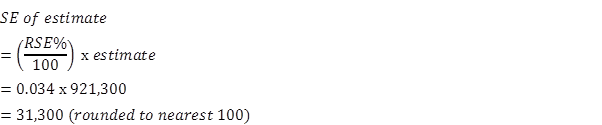7 Therefore, there are about two chances in three that the actual number of recent migrants as at November 2013 was in the range of 890,000 to 952,600 and about 19 chances in 20 that the value was in the range of 858,700 to 983,900. This example is illustrated in the diagram below: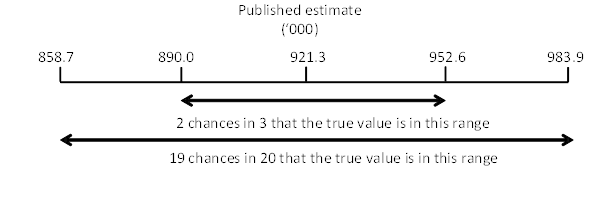PROPORTIONS AND PERCENTAGES 8 Proportions and percentages formed from the ratio of two estimates are also subject to sampling errors. The size of the error depends on the accuracy of both the numerator and the denominator. A formula to approximate the RSE of a proportion is given below. This formula is only valid when the numerator is a subset of the denominator.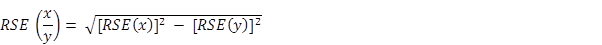9 As an example, using estimates from Table 1, of the 18,295,900 people aged 15 and over, 1.3%, or 245,400 people, had a skilled visa as at November 2013. The RSE for 245,400 is 5.9% and the RSE for 18,295,900 is 0.2% (see Table 1: RSE of Estimate). Applying the above formula, the RSE for the percentage of people who had a skilled visa as at November 2013 is: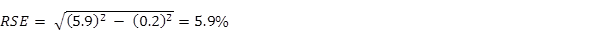10 Therefore, the SE for the proportion of people aged 15 and over who had a skilled visa as at November 2013 is 0.1 percentage points ((5.9/100) x 1.3 =0.1). Hence, there are about two chances in three that the proportion of people who had a skilled visa as at November 2013 is between 1.2% and 1.4%, and 19 chances in 20 that the proportion is between 1.1% and 1.5%. DIFFERENCES 11 Published estimates may also be used to calculate the difference between two survey estimates (numbers or proportions). Such an estimate is also subject to sampling error. The sampling error of the difference between two estimates depends on their SEs and the relationship (correlation) between them. An approximate SE of the difference between two estimates (x-y) may be calculated by the following formula:12 While this formula will only be exact for differences between separate and uncorrelated characteristics or sub populations, it provides a good approximation for the differences likely to be of interest in this publication. SIGNIFICANCE TESTING 13 A statistical significance test for any comparisons between estimates can be performed to determine whether it is likely that there is a difference between two corresponding population characteristics. The standard error of the difference between two corresponding estimates (x and y) can be calculated using the formula above. This standard error is then used to calculate the following test statistic: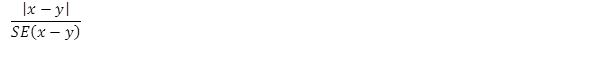14 If the value of this test statistic is greater than 1.96 then there is evidence, with a 95% level of confidence, of a statistically significant difference in the two populations with respect to that characteristic. Otherwise, it cannot be stated with confidence that there is a real difference between the populations with respect to that characteristic. OTHER SOURCES OF ERROR 15 The imprecision due to sampling variability, which is measured by the SE, should not be confused with inaccuracies that may occur because of imperfections in reporting by respondents and recording by interviewers, and errors made in coding and processing data. Inaccuracies of this kind are referred to as non-sampling error, and they occur in any enumeration, whether it be a full count or sample. Every effort is made to reduce non-sampling error to a minimum by careful design of questionnaires, intensive training and supervision of interviewers, and efficient operating procedures. Document Selection These documents will be presented in a new window.